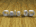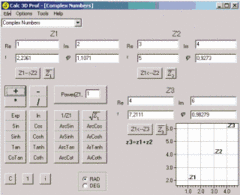Search
SoftwareCalc 3D is a collection of mathematical tools for highschool and university. The calculator can do statistics, best fits, function plotting, integration. It handles vectors, matrices, complex numbers, coordinates, regular polygons and intersections. For objects ( like point, line, plane and sphere) distances and intersections are calculated. Cartesian, spherical and cylindrical coordinates can be transformed into each other. Carthesian plot, polar plot, parametric plot, best fit, fast fourier transformation, histogram, smooth,...